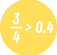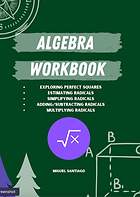top of page

There is no better way to improve your math survival skills than PRACTICE.

If you're is not feeling confident in a specific math topic, get a workbook and practice away!

It simple: search your math topic and get to practicing!

# Explore the Lands!

Explore your favorite land and find the workbook catered to you! Choose from a digital or physical copy. Happy Trails!Fractions & Decimals

Simplifying fractions & mixed numbers, adding/subtracting/multiplying/dividing fractions & decimals.

# Algebra CourtsMulti-Step Equations

1-2 Step equations, distributive property, using a divisor, combining like terms, and multi-step equations.# Lake Algebra 2System of Equations

Systems by Graphing, Substitution, Elimination, and Applications.System of Equations

Systems by Graphing, Substitution, Elimination, and Applications.Angle Relationships

Types of Angles, Exterior Angle Theorem, Parallel Lines, and Angles in Polygons.Right Triangle Geometry

Pythagorean theorem and its converse, special right triangles, using SOH CAH TOA

# Mt. CalculusBasic Integration and FTC

Power Rule, basic trig integration, Fundamental Theorem of Calculus, area under the curve.

Geomety
Calculus
Algebra2